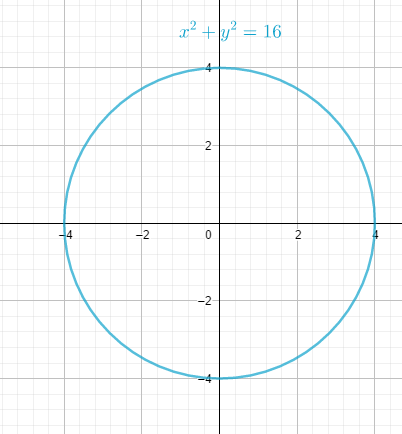### Math Notes

Subjects

#### Analytic Geometry Solutions

##### Topics || Problems

What is the graph of the equation $$18x^2 + 18 y^2 = 288$$
Solution:

The curve represents a circle at the origin, $$x^ +y^2 = r^2$$.

Simplify the equation to its standard form: $$18x^2 + 18y^2 = 288$$

$$x^2 + y^2 = 16$$

Locate the center and the radius of the circle.

The center of the circle is at the origin.

$$r^2 = 16$$

$$r = 4$$

Graph the curve.